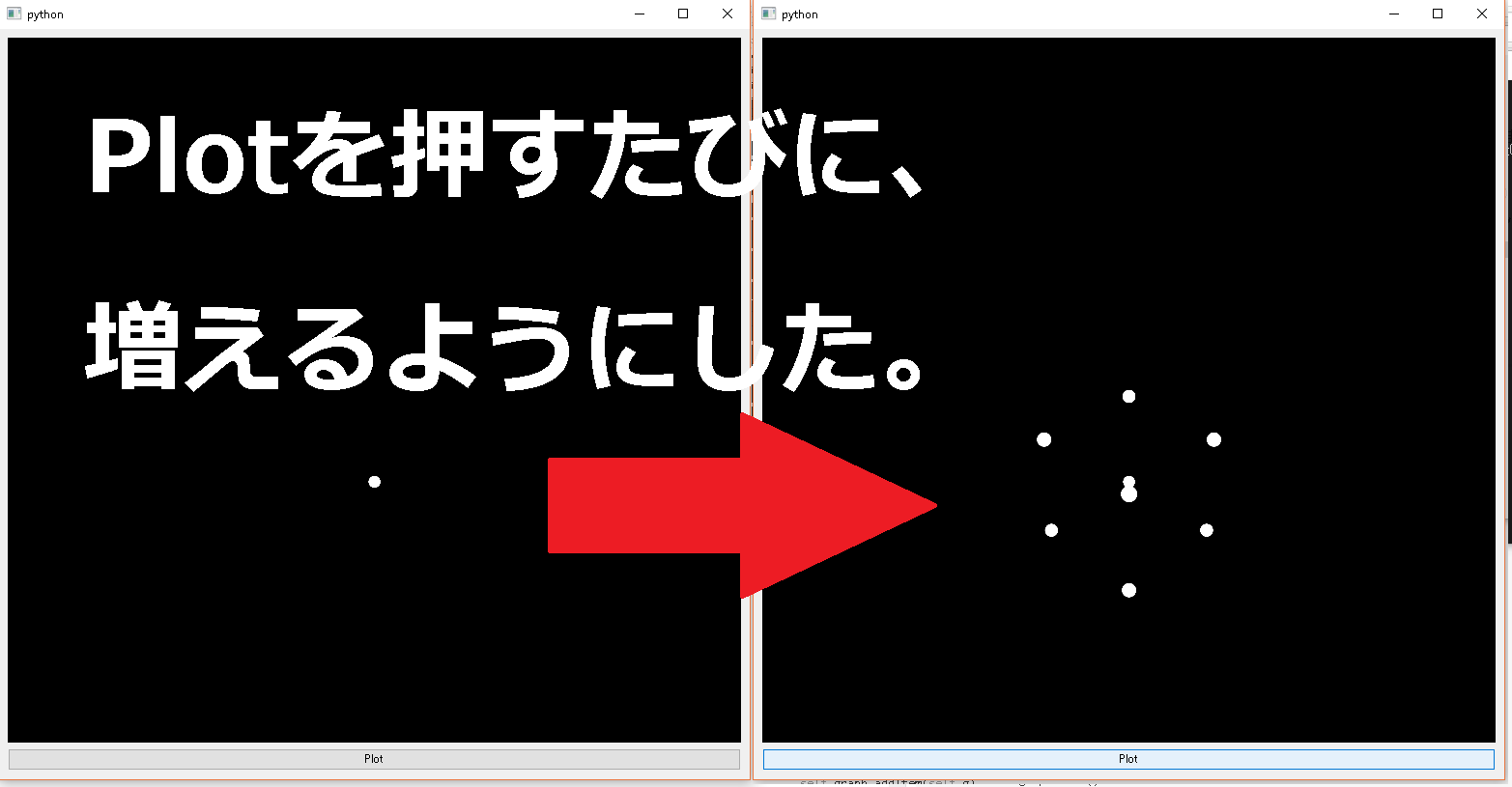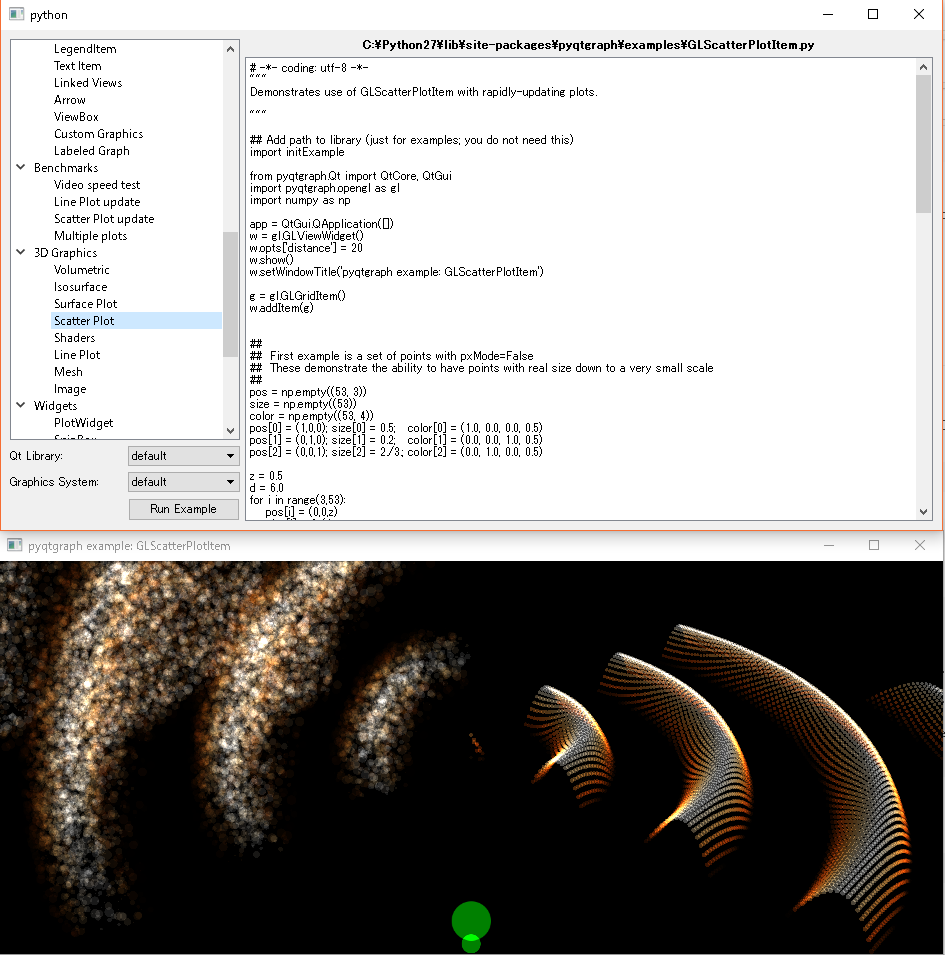# [PYTHON] 3D scatter plot with PyQtGraph

I tried to challenge the GUI of 3D plot using PyQtGraph. For those who have never done it, I thought it was a tough job, so I wrote it down. I did it on Windows.

I'll write the code first, but ** if you can't do it, read below. ** Maybe it just doesn't include OpenGL (pyopengl).

# For the time being

Save the following code as a file such as home a.py and execute it with python a.py. I intend to try it with as simple a structure as possible.

``````import sys
from PySide import QtCore, QtGui
import numpy as np
import pyqtgraph.opengl as gl
import pyqtgraph as pg

class Ui_MainWindow(object):
def setupUi(self, MainWindow):
MainWindow.setObjectName("MainWindow")
MainWindow.resize(777, 777)
self.centralwidget = QtGui.QWidget(MainWindow)
self.centralwidget.setObjectName("centralwidget")
MainWindow.setCentralWidget(self.centralwidget)

self.verticalLayout = QtGui.QVBoxLayout(self.centralwidget)
self.verticalLayout.setObjectName("verticalLayout")

self.graph = gl.GLViewWidget(self.centralwidget)
self.graph.setObjectName("graph")

self.graph.opts['distance'] = 30
self.graph.show()
self.g = gl.GLGridItem()

self.n=1
numX, startX, endX = self.n, -1, 1+self.n
numY, startY, endY = self.n, -1, 1+self.n
numZ, startZ, endZ = self.n, -1, 1+self.n

X = np.linspace(startX, endX, numX)
Y = np.linspace(startY, endY, numY)
Z = np.linspace(startZ, endZ, numZ)

#position of scatter in 3D
pos = np.array([[i,j,k] for i in X for j in Y for k in Z])

color = (1,1,1,1)
size = 0.5

self.scttrPlt = gl.GLScatterPlotItem(pos=pos, size=size, color=color, pxMode=False)
self.scttrPlt.translate(5,5,0)

self.psbtn = QtGui.QPushButton(self.centralwidget)
self.psbtn.setObjectName("psbtn")
self.psbtn.setText("Plot")

QtCore.QObject.connect(self.psbtn, QtCore.SIGNAL("clicked()"), self.plot)

def plot(self):
self.n+=1
numX, startX, endX = self.n, -1, 1+self.n
numY, startY, endY = self.n, -1, 1+self.n
numZ, startZ, endZ = self.n, -1, 1+self.n

X = np.linspace(startX, endX, numX)
Y = np.linspace(startY, endY, numY)
Z = np.linspace(startZ, endZ, numZ)

pos = np.array([[i,j,k] for i in X for j in Y for k in Z])
color = (1,1,1,1)
size = 0.5

self.scttrPlt.setData(pos=pos,color=color,size=size)

pg.setConfigOption('foreground', 'k')
pg.setConfigOption('background', 'w')

class ControlMainWindow(QtGui.QMainWindow):
def __init__(self, parent=None):
super(ControlMainWindow, self).__init__(parent)
self.ui = Ui_MainWindow()
self.ui.setupUi(self)

if __name__ == "__main__":
app = QtGui.QApplication(sys.argv)
mySW = ControlMainWindow()
mySW.show()
sys.exit(app.exec_())
``````# What you need OpenGL

• OpenGL
• PyQtGraph
• Python
• PySide

At the time of writing this, I don't know what OpenGL is. When using 3DCG software, it is recognized that a check box such as OpenGL appears.

``````python -m pyqtgraph.example
``````

Even if you select "3D graphics> Scatter plot" from the launcher of I only got an error. I get the following error.

``````E:\hoge>python -m pyqtgraph.examples
Using PySide (default graphics system)
Using PySide (default graphics system)
Traceback (most recent call last):
File "C:\Python27\lib\site-packages\pyqtgraph\examples\GLScatterPlotItem.py", line 11, in <module>
import pyqtgraph.opengl as gl
File "C:\Python27\lib\site-packages\pyqtgraph\opengl\__init__.py", line 1, in <module>
from .GLViewWidget import GLViewWidget
File "C:\Python27\lib\site-packages\pyqtgraph\opengl\GLViewWidget.py", line 2, in <module>
from OpenGL.GL import *
ImportError: No module named OpenGL.GL
``````

There is no module named OpenGL.GL ... Let's do the following on the command line. By the way, the name is not OpenGL, but pyopengl in all lowercase.

``````pip install pyopengl
``````
``````E:\hogehoge>pip install pyopengl
Collecting pyopengl
100% |################################| 1.2MB 298kB/s
Building wheels for collected packages: pyopengl
Running setup.py bdist_wheel for pyopengl ... done
Stored in directory: C:\Users\yusuke\AppData\Local\pip\Cache\wheels\1c\17\50\f69d63e0a8169fb890f5a167817a73391be85d30e86fd29504
Successfully built pyopengl
Installing collected packages: pyopengl
Successfully installed pyopengl-3.1.0
``````

This time, it's done.The image above is a sample that comes with PyQt Graph. It works in real time. You can also check the code from the sample launcher.

## pip must be the latest version

``````python -m pip install --upgrade pip
``````

let's do it. I didn't do it, so I got the following error.

``````E:\hoge>pip install pyopengl
Collecting OpenGL
Could not find a version that satisfies the requirement OpenGL (from versions: )
No matching distribution found for OpenGL
You are using pip version 8.1.2, however version 9.0.1 is available.
You should consider upgrading via the 'python -m pip install --upgrade pip' command.
``````